Question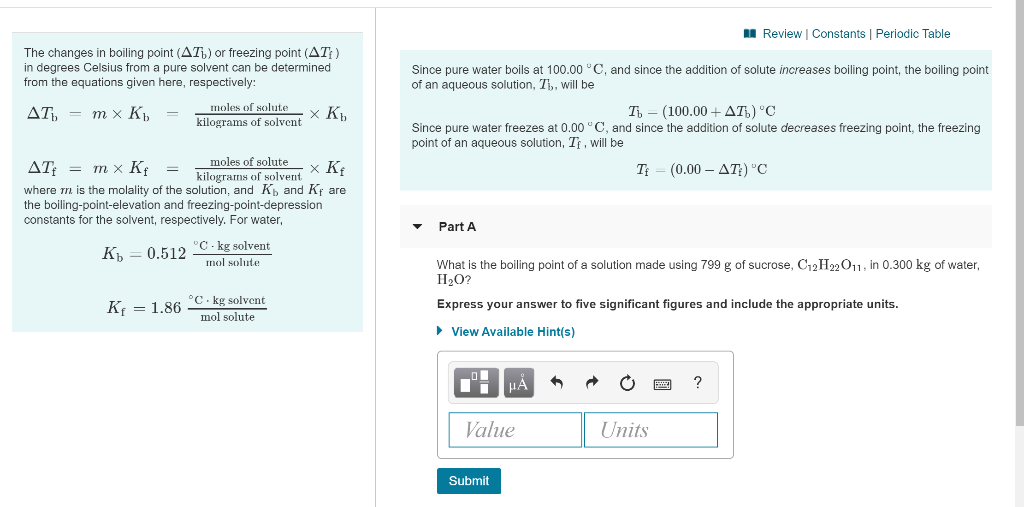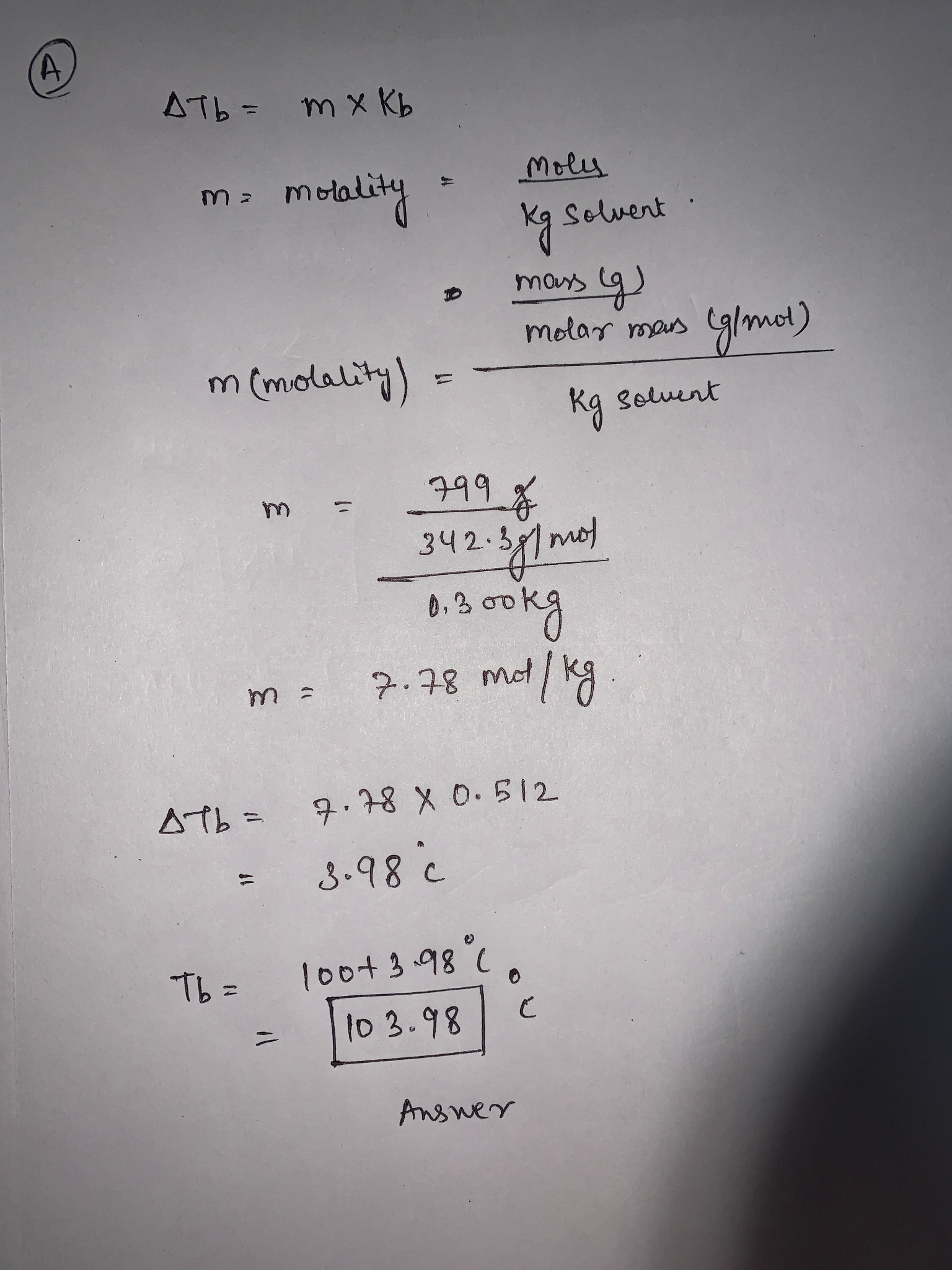#### Earn Coins

Coins can be redeemed for fabulous gifts.

Similar Homework Help Questions
• ### Review Constants Periodic Table The changes in boiling point (AT) or freezing point (AT) in degrees...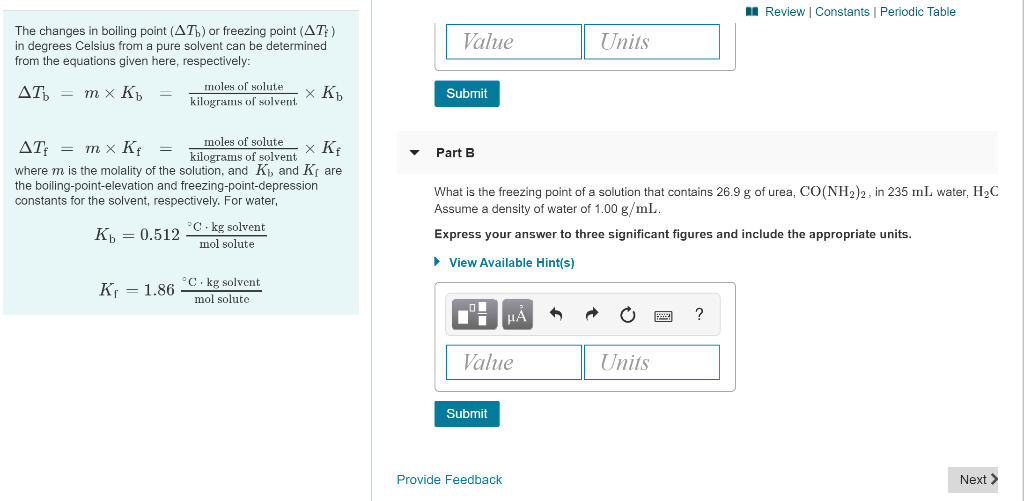Review Constants Periodic Table The changes in boiling point (AT) or freezing point (AT) in degrees Celsius from a pure solvent can be determined from the equations given here, respectively: Value Units moles of solute AT = mx Kb = 7 Submit kilograms of solvent XRb moles of solutex Kf Part B AT: = mx Kf = kilograms of solvent where m is the molality of the solution, and K and K the boiling-point-elevation and freezing-point-depression constants for the solvent,...

• ### Molality, Freezing Point, and Boiling Point 29 of 44 - Part 3 Review Constants Periodic Table...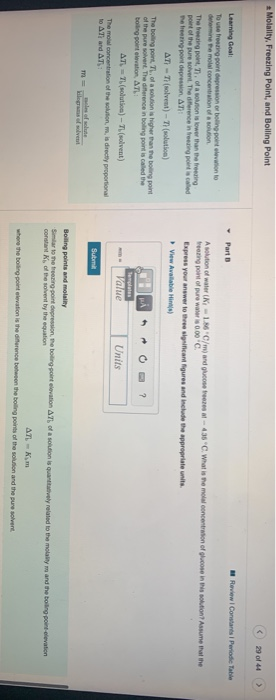Molality, Freezing Point, and Boiling Point 29 of 44 - Part 3 Review Constants Periodic Table Learning Goal Toute ring point depression or boling point elevation to din Pemola concentration of a solution The bring point, T. of a sortion is lower than the freezing point of the pure solvent. The difference in freezing point is called The treening point depression. AT AT - (solvent) - Tolution) The big pont, Th. of a solution is higher than the boting point...

• ### Calculate the freezing point of a 11.50 m aqueous solution of propanol. Freezing point constants can...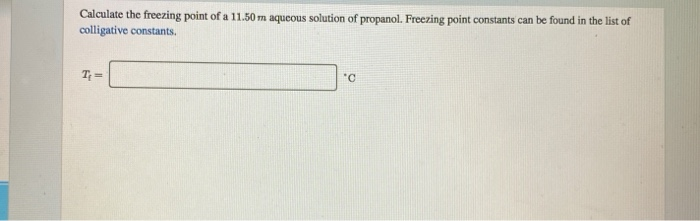Calculate the freezing point of a 11.50 m aqueous solution of propanol. Freezing point constants can be found in the list of colligative constants. Colligative Constants Constants for freezing point depression and boiling point elevation calculations at 1 atm: Solvent Formula Kvalue" Normal freezing ky value Normal bolling (°C/m) point (°C) (°C/m) point (°C) H20 1.86 0.00 0.512 100.00 CGHS 5.12 5.49 CH12 20.8 6.59 CyH60 1.99 -117.3 calu 29.8 -22.9 76.8 water benzene cyclohexane ethanol carbon tetrachloride camphor 2.53...

• ### part b and c please ( 5 of 15 A Review | Constants Periodic Table Part...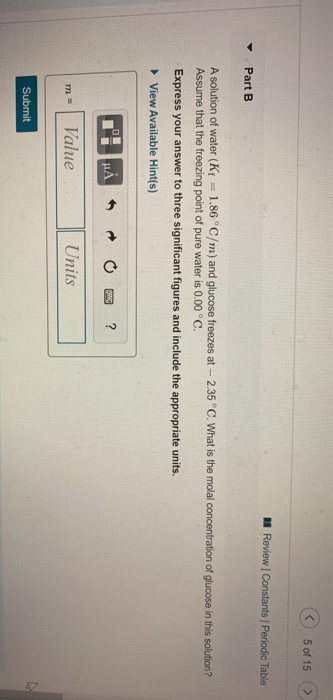part b and c please ( 5 of 15 A Review | Constants Periodic Table Part B A solution of water (Kf = 1.86°C/m) and glucose freezes at -- 2.35 °C. What is the molal concentration of glucose in this solution? Assume that the freezing point of pure water is 0.00 °C. Express your answer to three significant figures and include the appropriate units. View Available Hint(s) T'I HAO ? m= Value Units Submit A Review Constants Periodic Table AT)...

• ### Enter your answer in the provided box. The formula that governs the depression of freezing point...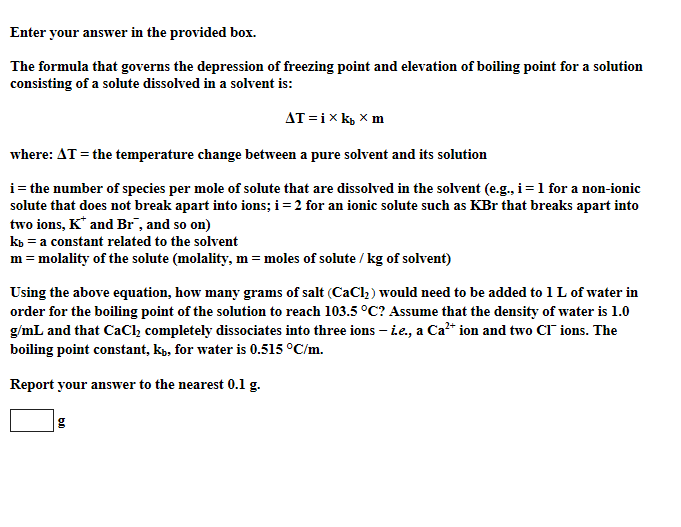Enter your answer in the provided box. The formula that governs the depression of freezing point and elevation of boiling point for a solution consisting of a solute dissolved in a solvent is: where:AT = the temperature change between a pure solvent and its solution i = the number of species per mole of solute that are dissolved in the solvent (e.g., i = 1 for a non-ionic solute that does not break apart into ions, i = 2 for...

• ### A solution is made by dissolving 0.749 mol of nonelectrolyte solute in 861 g of benzene....

A solution is made by dissolving 0.749 mol of nonelectrolyte solute in 861 g of benzene. Calculate the freezing point, Tf, and boiling point, Tb, of the solution. Constants may be found here. Solvent Formula Kf value* (°C/m) Normal freezing point (°C) Kb value (°C/m) Normal boiling point (°C) water H2O 1.86 0.00 0.512 100.00 benzene C6H6 5.12 5.49 2.53 80.1 cyclohexane C6H12 20.8 6.59 2.92 80.7 ethanol C2H6O 1.99 –117.3 1.22 78.4 carbon tetrachloride   CCl4 29.8 –22.9 5.03 76.8...

• ### a Calculate the freezing point and the boiling point of each of the following aqueous solutions....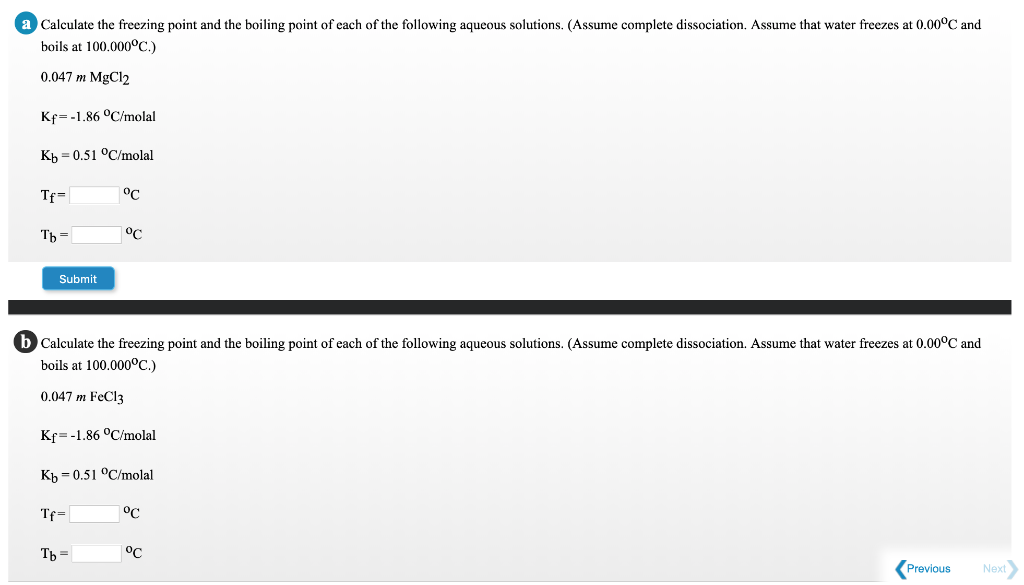a Calculate the freezing point and the boiling point of each of the following aqueous solutions. (Assume complete dissociation. Assume that water freezes at 0.00°C and boils at 100.000ºC.) 0.047 m MgCl2 Kr=-1.86 °C/molal Kb =0.51 °C/molal Tf= °C Tb = °C Submit b Calculate the freezing point and the boiling point of each of the following aqueous solutions. (Assume complete dissociation. Assume that water freezes at 0.00°C and boils at 100.000°C.) 0.047 m FeCl3 Kf=-1.86 °C/molal Kb =0.51 °C/molal...

• ### Freezing points are lowered as a function of the number of moles of solute particles per...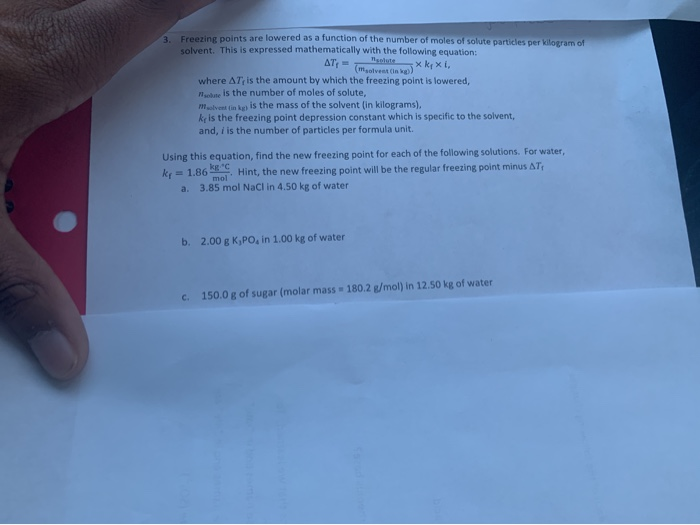Freezing points are lowered as a function of the number of moles of solute particles per kilogram of solvent. This is expressed mathematically with the following equation ATt Xkxi (mgolvending) where AT, is the amount by which the freezing point is lowered, Toute is the number of moles of solute, m ening is the mass of the solvent (in kilograms). ke is the freezing point depression constant which is specific to the solvent, and, i is the number of particles...

• ### Enter your answer in the provided box. The formula that governs the depression of freezing point...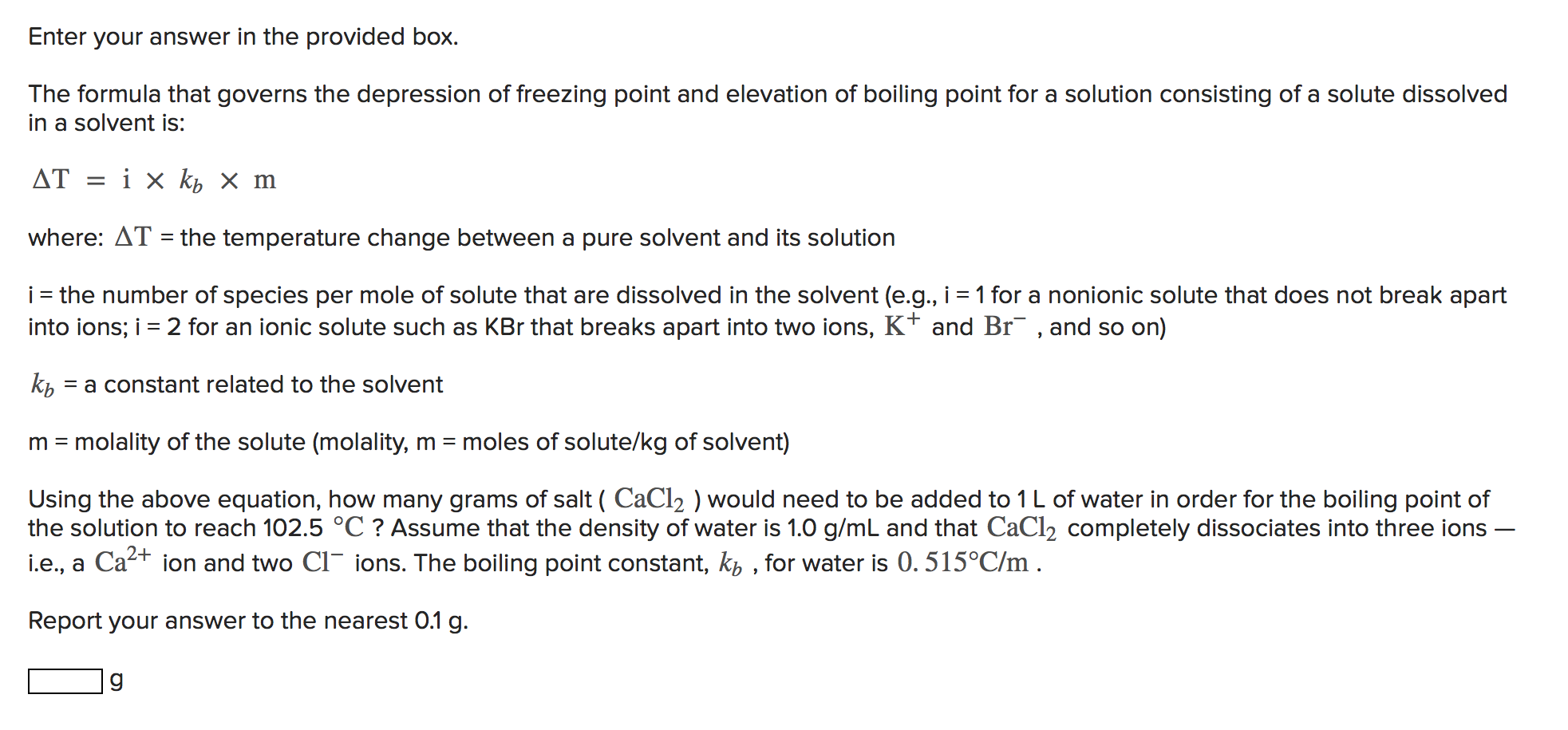Enter your answer in the provided box. The formula that governs the depression of freezing point and elevation of boiling point for a solution consisting of a solute dissolved in a solvent is: AT = i x kb x m where: AT = the temperature change between a pure solvent and its solution i = the number of species per mole of solute that are dissolved in the solvent (e.g., i = 1 for a nonionic solute that does not...

• ### 1h. A certain pure solvent freezes at 39.8°C and has a freezing point depression constant Kf...

1h. A certain pure solvent freezes at 39.8°C and has a freezing point depression constant Kf = 0.777°C/m. What is the predicted freezing point (in °C) of a solution made from this solvent that is (1.90x10^0) m in a non-electrolyte solute? 1i. When (8.23x10^1) g of a non-electrolyte is dissolved in (5.2600x10^2) g of a solvent (with Kb = 0.416°C/m) the boiling point of the solution is 1.50°C higher than the boiling point of the pure solvent. What is the...